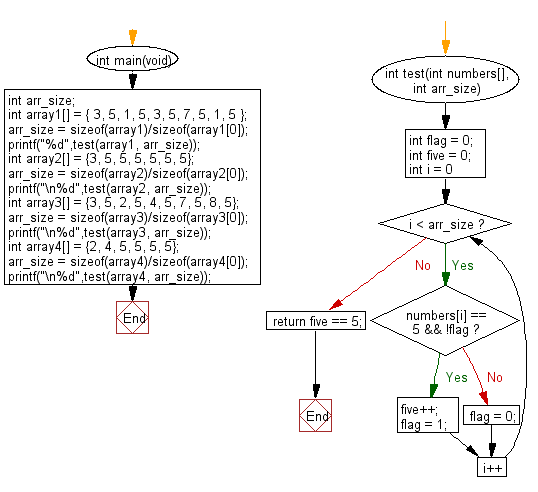﻿ C : 5 appears 5 times in an array and no 5 is adjacent# C Exercises: Check a given array of integers and return true if the value 5 appears 5 times and there are no 5 next to each other

## C-programming basic algorithm: Exercise-64 with Solution

Write a C program to check a given array of integers and return true if the value 5 appears 5 times and there are no 5 next to each other.

C Code:

``````#include <stdio.h>
#include <stdlib.h>
int main(void){
int arr_size;
int array1[] = { 3, 5, 1, 5, 3, 5, 7, 5, 1, 5 };
arr_size = sizeof(array1)/sizeof(array1);
printf("%d",test(array1, arr_size));
int array2[] = {3, 5, 5, 5, 5, 5, 5};
arr_size = sizeof(array2)/sizeof(array2);
printf("\n%d",test(array2, arr_size));
int array3[] = {3, 5, 2, 5, 4, 5, 7, 5, 8, 5};
arr_size = sizeof(array3)/sizeof(array3);
printf("\n%d",test(array3, arr_size));
int array4[] = {2, 4, 5, 5, 5, 5};
arr_size = sizeof(array4)/sizeof(array4);
printf("\n%d",test(array4, arr_size));
}

int test(int numbers[], int arr_size)
{
int flag = 0;
int five = 0;

for (int i = 0; i < arr_size; i++)
{
if (numbers[i] == 5 && !flag)
{
five++;
flag = 1;
}
else
{
flag = 0;
}
}

return five == 5;
}
``````

Sample Output:

```1
0
1
0
```

Pictorial Presentation:Flowchart:C Programming Code Editor:

What is the difficulty level of this exercise?

Test your Programming skills with w3resource's quiz.

﻿

## C Programming: Tips of the Day

Why doesn't a+++++b work?

printf("%d",a+++++b); is interpreted as (a++)++ + b according to the Maximal Munch Rule!.

++ (postfix) doesn't evaluate to an lvalue but it requires its operand to be an lvalue.

! 6.4/4 says the next preprocessing token is the longest sequence of characters that could constitute a preprocessing token"

Ref : https://bit.ly/3fdldUT